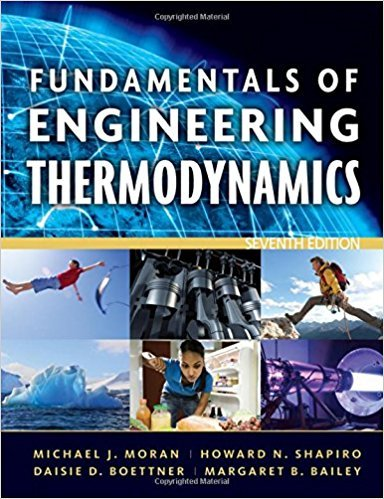×
Get Full Access to Fundamentals Of Engineering Thermodynamics - 7 Edition - Chapter 6 - Problem 43p
Get Full Access to Fundamentals Of Engineering Thermodynamics - 7 Edition - Chapter 6 - Problem 43p

×

# Answer: Applying the Entropy Balance: Closed SystemsAirISBN: 9780470495902 50

## Solution for problem 43P Chapter 6

Fundamentals of Engineering Thermodynamics | 7th Edition

• Textbook Solutions
• 2901 Step-by-step solutions solved by professors and subject experts
• Get 24/7 help from StudySoup virtual teaching assistantsFundamentals of Engineering Thermodynamics | 7th Edition

4 5 1 280 Reviews
21
2
Problem 43P

Problem 43P

Applying the Entropy Balance: Closed Systems

Air contained in a rigid, insulated tank fitted with a paddle wheel, initially at 300 K, 2 bar, and a volume of 2 m3, is stirred until its temperature is 500 K. Assuming the ideal gas model for the air, and ignoring kinetic and potential energy, determine (a) the final pressure, in bar, (b) the work, in kJ, and (c) the amount of entropy produced, in kJ/K. Solve using

(a) data from Table A-22.

(b) constant cv read from Table A-20 at 400 K.

Compare the results of parts (a) and (b).

Table A-20 Ideal Gas Specific Heats of Some Common Gases (kJ/kg · K)Table A-22 Ideal Gas Properties of AirStep-by-Step Solution:

a.)

Step 1 of  4

We have to find the final pressure, work done and  the amount of entropy produced,for the air contained in a rigid, insulated tank fitted with a paddle wheel, initially at 300 K, 2 bar, and a volume of 2 m3 is stirred until its temperature is 500 K.

The final pressure can be found using ideal gas equationNow,andSo,Hence,== 2= 3.33 bar

Therefore, the final pressure is 3.33 bar .

Step 2 of 4

Step 3 of 4

##### ISBN: 9780470495902

This textbook survival guide was created for the textbook: Fundamentals of Engineering Thermodynamics, edition: 7. The full step-by-step solution to problem: 43P from chapter: 6 was answered by , our top Engineering and Tech solution expert on 07/20/17, 09:01AM. This full solution covers the following key subjects: Table, air, ideal, gas, entropy. This expansive textbook survival guide covers 14 chapters, and 1501 solutions. The answer to “Applying the Entropy Balance: Closed SystemsAir contained in a rigid, insulated tank fitted with a paddle wheel, initially at 300 K, 2 bar, and a volume of 2 m3, is stirred until its temperature is 500 K. Assuming the ideal gas model for the air, and ignoring kinetic and potential energy, determine (a) the final pressure, in bar, (b) the work, in kJ, and (c) the amount of entropy produced, in kJ/K. Solve using(a) data from Table A-22.________________(b) constant cv read from Table A-20 at 400 K.Compare the results of parts (a) and (b).Table A-20 Ideal Gas Specific Heats of Some Common Gases (kJ/kg · K) Table A-22 Ideal Gas Properties of Air” is broken down into a number of easy to follow steps, and 113 words. Fundamentals of Engineering Thermodynamics was written by and is associated to the ISBN: 9780470495902. Since the solution to 43P from 6 chapter was answered, more than 600 students have viewed the full step-by-step answer.

Unlock Textbook Solution